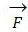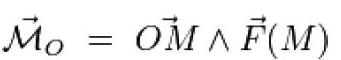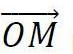# TORQUE MEASUREMENT

## Definitions

Torque measurement is based on the fundamental notion of the moment of a force, defined as follows (cf. fig. 1):

The moment of force, where M is its application point, in relation to point O, is defined by:It is a pseudovectorproduced by the vectorial product of vectorby vector force. It is applied to O, perpendicularly to the plane containing the vector force and point O, and its intensity is equal to the product of the intensity of force F by length OH of the lowered perpendicular of point O in the direction of the force.

A system of two parallel non co-linear forces of equal intensity and opposite direction constitutes a ‘torque’ of forces. The moment of such a torque is equal to the sum of the moments of each of the forces. Its intensity is therefore equal to the product of intensity F, common to both forces, by distance D of both forces, measured perpendicularly to their action line.It is necessary to always define if we are in the presence of the moment of a force or of a torque because the conditions of their good measurement are very different.

## Torque meter: designed for torsion

A torque meter is a measurement instrument analogue to a dynamometer, designed to operate in torsion. Its proof body has a cylindrical shape, subject to shearing strains measured by strain gauges. This type of strain provides measurements which are exactly proportional to the torque applied to the transducer.

As for a dynamometer, the torque meter is linked to a measurement bridge allowing it to feed the transducer and to process the transmitted signal.

## Measuring with a torque meter

• ### Two applications for a torque meter:

A torque meter can be used for two operations:

• pure torque measurement
• calibration or verification of a torque wrench
• ### A torque meter is subject to two main torques

The operation conditions required for a torque meter are more difficult to achieve than those needed for a dynamometer. In fact, a torque meter is subject to two main torques: the first comes from the torque being measured and the second from the reaction torque preventing the torque meter from turning around its axis. These two torques are joined by interfering torques and efforts arising from failures in the application devices of the two main torques.

## Quality of torque meter measurement

The quality of measurement mainly depends on the alignment of three axes:

• the axis of the torque pseudovector being measured
• the axis of the reaction torque pseudovector
• the torque meter axis

For this to happen, all three axes must be materialised by means of mechanical elements which are centred and aligned in relation to one another.

## Avoiding torque meter measurement errors Alignment failures

Alignment failures cause interfering moments perpendicular to the torque meter axis, which induce measurement errors varying by several percentage points from the torque measurement performed.

The interfering torques can be reduced significantly by means of specific connecting mechanical devices which limit the effect of alignment failures. These are cardan joints, elastic blades or discs. Rexnord coupling devices are particularly effective.

NOTE : Spanish version /en/tecnologia/esfuerzo-de-torsion/medicion-del-par